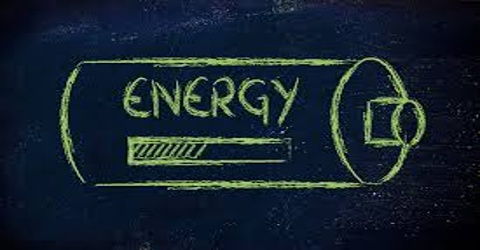# Energy Definition with Types

When a body can work, it is considered that it has energy. Energy is measured by the total amount of work that a body can do. That means, by work done we can measure energy. When a body does work its energy decreases and the body on which work is done its energy increases. Energy has no mass, weight and volume. The body which has less capacity to do work has less energy. So, it can be said that work is the measure of energy. If it is said that a body has done W amount of work, then it is understood that energy used by it is W. When a body does work against the force then it loses energy. Again, if work is done on a body by the force, then it gains energy.

Definition: Energy of a body is the capacity or ability to do work. It is measured by the amount of work the body can do. Like work energy is also a scalar quantity.

Amount of work = work done = applied force x displacement of point of action of the force.

Petrol vapour in a motor engine, water vapour pressure drives piston in a vapour engine. So, vapour has energy. Again, electricity has energy. Trains, factories and industries are run by electricity. The universe is moving because there is energy. Energy can be transformed, but can never be created nor destroyed. So, in transformation process total energy remains unchanged. We will learn in detail about it in conservation principle of energy. Energy has different forms, viz-

• Mechanical energy
• Heat energy
• Light energy
• Sound energy
• Magnetic energy
• Electrical energy
• Chemical energy
• Nuclear energy
• Solar energy